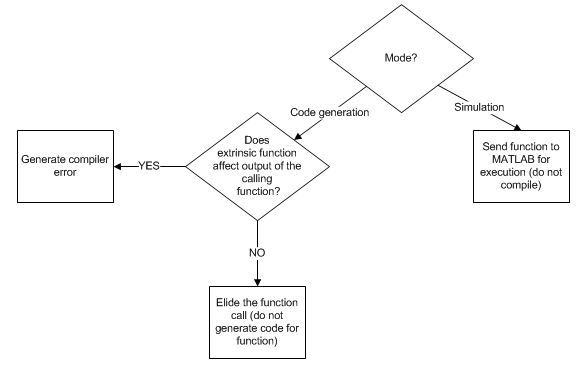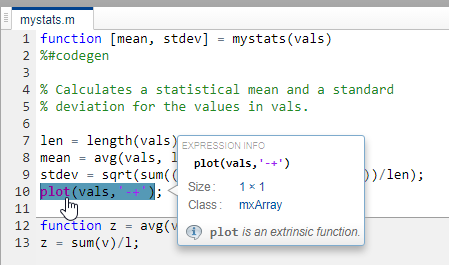## 使用 MATLAB 引擎在生成的代码中执行函数调用

`coder.extrinsic('foo')` 指令包含在某特定 MATLAB 函数中会将该 MATLAB 函数中对 `foo` 的所有调用都声明为外部调用。或者，您可能希望将外部声明的范围缩小到仅对 `foo` 的一次调用。请参阅使用 feval 调用 MATLAB 函数### 将函数声明为外部函数的情形

• 函数执行显示或记录操作。此类函数主要在仿真期间有用，在嵌入式系统中不使用。

• 在您的 MEX 执行或 Simulink 仿真中，您要使用代码生成不支持的 MATLAB 函数。此工作流不适用于非仿真目标。

• 您使用 `coder.const` 指示代码生成器对函数调用进行常量折叠。在这种情况下，仅当 MATLAB 引擎可用于执行调用时，才会在代码生成期间调用该函数。

### 使用 `coder.extrinsic` 构造

`coder.extrinsic('foo')`

• 在调用函数之前将其声明为外部函数。

• 不要在条件语句中使用外部声明。

• 将外部函数的返回值赋给已知类型。请参阅使用 mxArray#### 外部函数声明的作用域

`coder.extrinsic` 构造具有函数作用域。以如下代码为例：

```function y = foo %#codegen coder.extrinsic('rat','min'); [N D] = rat(pi); y = 0; y = min(N, D); ```

• 在局部函数中将 MATLAB 函数声明为外部函数，如以下示例中所示：

```function y = foo %#codegen coder.extrinsic('rat'); [N D] = rat(pi); y = 0; y = mymin(N, D); function y = mymin(a,b) coder.extrinsic('min'); y = min(a,b); ```

这里，函数 `rat` 每次在主函数 `foo` 内调用时都是外部函数，但函数 `min` 只有在局部函数 `mymin` 内调用时才是外部函数。

• 请使用 `feval` 调用 MATLAB 函数，而不是使用 `coder.extrinsic` 构造。下一节将介绍这种方法。

#### 对非静态方法进行外部声明

• 将对 `foo` 的调用编写为函数调用。不要使用圆点表示法来编写调用。

• 使用语法 `coder.extrinsic('foo')``foo` 声明为外部函数。

```classdef myClass properties prop = 1 end methods function y = foo(obj,x) y = obj.prop + x; end end end ```

```function y = myFunction(x) %#codegen coder.extrinsic('foo'); obj = myClass; y = foo(obj,x); end ```

#### 其他用途

• 调用在仿真过程中不会生成输出，从而不会生成不必要代码的 MATLAB 函数。

• 使您的代码具有自说明，从而更容易调试。您可以扫描 `coder.extrinsic` 语句的源代码以隔离对 MATLAB 函数的调用，它们可能会创建并传播 `mxArrays`。请参阅使用 mxArray

### 使用 `feval` 调用 MATLAB 函数

```function y = foo coder.extrinsic('rat'); [N D] = rat(pi); y = 0; y = feval('min',N,D); ```

### 使用 mxArray

• 在变量中存储 `mxArray`

• `mxArray` 传递给外部函数。

• 将函数中的 `mxArray` 返回到 MATLAB。

• 运行时将 `mxArray` 转换为已知类型。请将 `mxArray` 赋给变量，此变量的类型已由以前的赋值定义。请参阅以下示例。

#### 将 mxArray 转换为已知类型

```function y = foo %#codegen coder.extrinsic('rat'); [N D] = rat(pi); y = min(N,D); ```

`codegen foo`
`Code generation successful.`

```Function output 'y' cannot be an mxArray in this context. Consider preinitializing the output variable with a known type. ```

```function y = foo %#codegen coder.extrinsic('rat'); [N D] = rat(pi); y = 0; % Define y as a scalar of type double y = min(N,D);```

`codegen -config:lib foo`
```??? The extrinsic function 'min' is not available for standalone code generation. It must be eliminated for stand-alone code to be generated. It could not be eliminated because its outputs appear to influence the calling function. Fix this error by not using 'min' or by ensuring that its outputs are unused. Error in ==> foo Line: 4 Column: 5 Code generation failed: View Error Report Error using codegen```

### 使用外部函数的限制

• 用来检查调用方或读取/写入调用方工作区的 MATLAB 函数在代码生成过程中不起作用。此类函数包括：

• 如果您的外部函数在运行时执行以下操作，则生成的代码中的函数可能会产生不可预知的结果：

• 更改文件夹

• 更改 MATLAB 路径

• 删除或添加 MATLAB 文件

• 更改警告状态

• 更改 MATLAB 预设

• 代码生成器不支持使用 `coder.extrinsic` 来调用位于私有文件夹中的函数。
• 代码生成器不支持使用 `coder.extrinsic` 来调用局部函数。